# TEE I

• where r is the radius of the cylinder or vessel and L is the distance between the two point (or the length of the cylinder or vessels).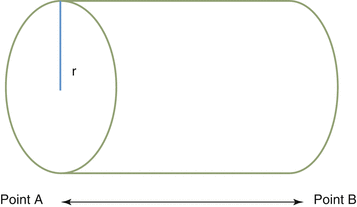Fig. 52.1
Illustration of a cylinder with parameters used in calculating area and flow across two points in the cylinder where r is cross-sectional radius of cylinder and L is distance between point A and point B 

• Also, flow rate (Q) is calculated as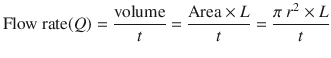• where t is time for fluid to traverse from point A to B.

• Using the law of conservation of mass (continuity equation) and assuming laminar flow,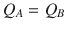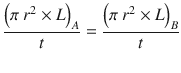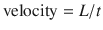• Therefore, the formula can be simplified to read;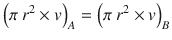• The velocity (and distance L) that blood travels in a blood vessel can be measured by TEE using continuous or pulse wave Doppler. Continuous wave uses two crystals; one crystal continuously transmits ultrasound wave and the other crystal continuously receives ultrasound waves thus allowing for measurement of high-frequency Doppler velocities along the entire length of the ultrasound beam . In contrast to continuous wave measurement, pulse wave measurements use one ultrasound crystal for both transmitting and receiving ultrasound waves. This allows for measurement of low-frequency Doppler velocity (v) from a specific region of blood flow.

• Using either continuous or pulse wave Doppler allows for the measurement of velocity (v) and distance (measured by the echo machine as velocity time integral (VTI)). The VTI and v are obtained by tracing the area under the Doppler signal obtained. The VTI is also called the stroke distance that is the distance travelled by the sampled volume per heartbeat. Therefore, the pulse wave VTI is the distance travelled by a blood sample at a specific point in the LVOT, while the continuous wave VTI (usually measured across the aortic valve) is the longest distance travelled by blood across the aortic valve .

Sep 23, 2017 | Posted by in Uncategorized | Comments Off on TEE I## Full access? Get Clinical TreeGet Clinical Tree app for offline access